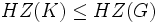# Cocentrality is transitive

This article gives the statement, and possibly proof, of a subgroup property (i.e., cocentral subgroup) satisfying a subgroup metaproperty (i.e., transitive subgroup property)
View all subgroup metaproperty satisfactions | View all subgroup metaproperty dissatisfactions |Get help on looking up metaproperty (dis)satisfactions for subgroup properties
Get more facts about cocentral subgroup |Get facts that use property satisfaction of cocentral subgroup | Get facts that use property satisfaction of cocentral subgroup|Get more facts about transitive subgroup property

## Statement

### Statement with symbols

If$H$ is a cocentral subgroup of$K$, and$K$ is a cocentral subgroup of$G$, then$H$ is a cocentral subgroup of$G$.

## Definitions used

### Cocentral subgroup

Further information: Cocentral subgroup

A subgroup$H$ of a group$G$ is termed cocentral in$G$ if$HZ(G) = G$, where$Z(G)$ is the center of$G$.

## Proof

Given: A group$G$, a cocentral subgroup$K$ of$G$, a cocentral subgroup$H$ of$K$. In other words,$HZ(K) = K$, and$KZ(G) = G$.

To prove:$H$ is cocentral in$G$, i.e.,$HZ(G) = G$.

Proof: Consider$C_G(Z(K))$. Clearly,$Z(G) \le C_G(Z(K))$, and$K \le C_G(Z(K))$, because$Z(K)$ centralizes$K$. Thus,$KZ(G) \le C_G(Z(K))$, so$C_G(Z(K)) = G$. Thus,$Z(K) \le Z(G)$. Thus,$HZ(K) \le HZ(G)$, so$K \le HZ(G)$, and thus$G = KZ(G) \le HZ(G)$. Thus, <mathG = HZ(G)[/itex].